# Understanding Precomputed Interest LoansCindy Fisher | September 10, 2020

Precomputed interest loans are a popular method of lending for borrowers requesting less than a few thousand dollars for a loan term of less than five years. They can be for more than that amount, but they are most common in short-term, small-dollar loans.

Precomputed loans use a method known as “rule of 78s” to calculate interest charges on a loan. The pre-calculated interest charges favor the lender over the borrower for short-term loans or if a loan is paid off early.

According to Investopedia, “rule of 78 requires the borrower to pay a greater portion of interest in the earlier part of a loan cycle, which decreases the potential savings for the borrower in paying off their loan.”

In some ways, precomputed loans are very much like non-revolving, simple-interest loans. Should the borrower take the full amount of months to pay off the loan, there would be no difference in the amount of interest paid by the borrower between a precomputed loan (which we call payment method 3) or a daily simple-interest loan (which we call payment method 6).

However, should the borrower make increased payments and pay off the loan early, the amount of interest paid weighs slightly more in the lender's favor. Here’s why…

Calculating Precomputed Loans

The rule of 78 calculation adds up the total amount of interest if the loan were paid off by the full term of the loan.

With daily simple-interest, interest is accrued daily. But with precomputed loans, all the interest that would be owed for the entire term is calculated up front when the loan is opened. The amount of precomputed interest can be found in the Original Unearned Interest field on the Loans > Account Information > Precomputed Loans screen, as shown below: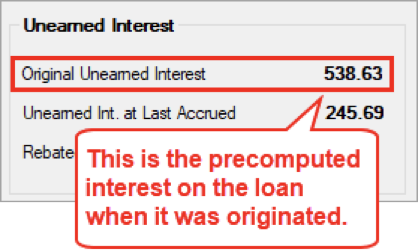In a 12-month loan a lender would sum the number of digits through 12 months in the following calculation:

1+2+3+4+5+6+7+8+9+10+11+12=78

In an 18-month loan, the sum of digits would be:

1+2+3+4+5+6+7+8+9+10+11+12+13+14+15+16+17+18=171

For a two-year loan the total sum of the digits would be 300, and so forth.

Once the sum of the months is calculated, the interest is weighted in reverse order applying greater weight to the earlier months. For a one-year loan the weighting factor would be 12/78 of the total interest in the first month; 11/78 in the second month; 10/78 in the third month; etc. For a two-year loan the weighting factor would be 24/300 in the first month; 23/300 in the second month; 22/300 in the third month, etc.

As an example, let’s assume a \$2,000 loan with precomputed interest calculated at 15 percent for a term of one year.

Let’s say the interest calculation method is set to 360/360, which means monthly interest would be calculated as follows:

Principal Balance X Interest Rate / 360 X 30 = Monthly Interest

2,000 X .15 / 360 X 30 = \$25

Then you would repeat that calculation for each month, assuming a minimum payment amount is made each month and the principal balance goes down by the amount of the P/I Constant going toward principal.

Quick tip: You can enter that information on the Loans > Account Information > Amortization Schedule screen to calculate the regular monthly interest amount of a loan, as shown below: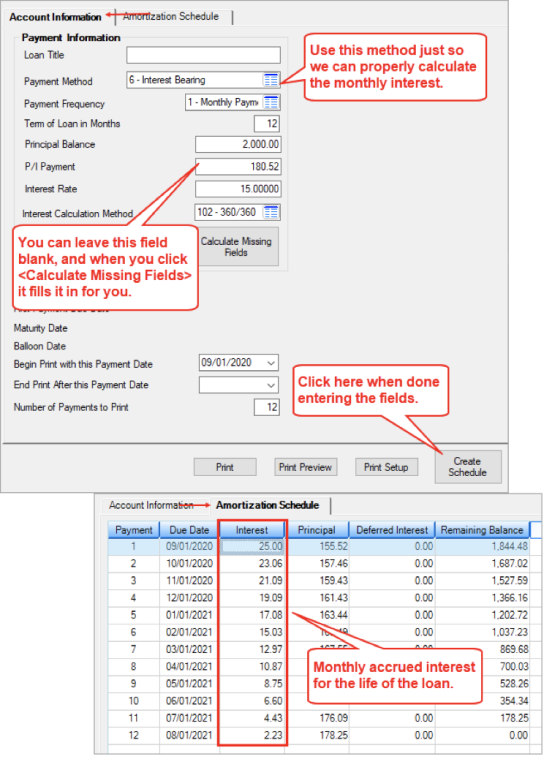Should the account owner take the full term to pay off the loan, they would pay \$166.20 of interest for the \$2,000 loan.

So now that we know that, we can calculate the precomputed interest, as shown in the following table: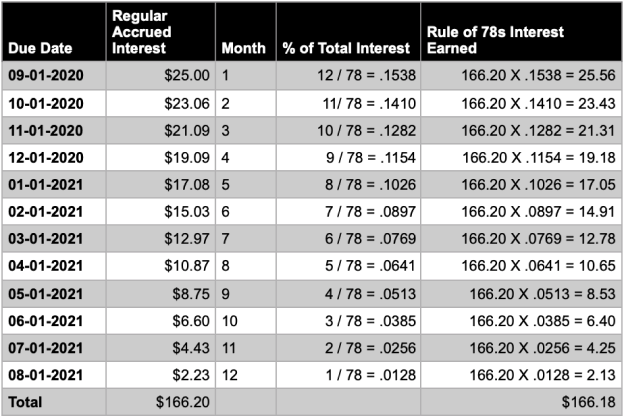Rebated Precomputed Interest

However, if the account owner pays off the loan early, a portion of the precomputed interest is rebated back to the borrower at payoff. This is considered “unearned interest,” as your institution hasn’t “earned” it yet.

Your institution earns the interest each monthend, similar to amortization. It earns that month’s accrual of precomputed interest.

Using the table above, let’s say a borrower pays off the loan on 01-01-2021. The system has earned four months of interest totaling \$89.48. The system subtracts earned interest from the original unearned interest to get the amount of interest rebated back to the borrower. So in this case, the interest amount rebated back to the borrower would be \$76.70 (166.18 – 89.48).

The Precomputed Loans screen in CIM GOLD shows this information, see below: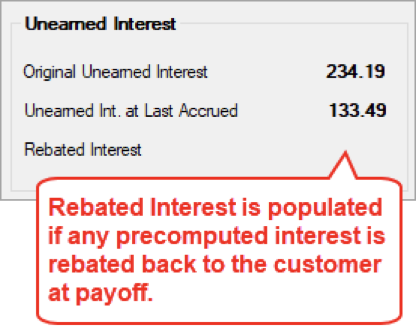Note: There’s more information to learn about precomputed loans and payoffs. We’ll discuss that in a future blog post.

P/I Constant

One thing to note about precomputed loans is that the P/I Constant is really just going completely to principal because the interest is included up front and totaled in with the loan. The principal balance on the loan is the amount of the loan plus all the precomputed interest (and all add-on insurance, amortizing fees, etc.). It’s just one total amount.

So when you view a precomputed loan on the Amortization Schedule screen, you’ll see Principal shows the total amount, with nothing going to Interest.

Example: The \$2,000 loan with 166.18 in precomputed interest means the Principal Balance is now \$2,166.18. Divide that by 12 months of payments means the P/I Constant is \$180.52.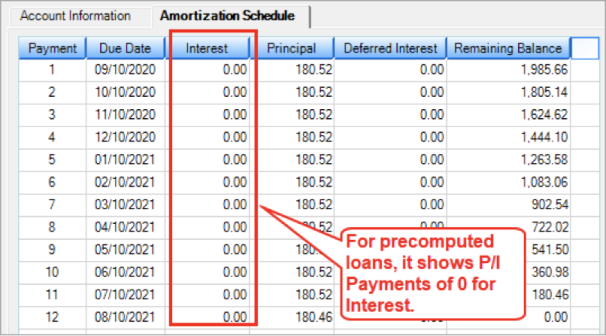Cindy Fisher | September 10, 2020

Subscribe to Our Blog